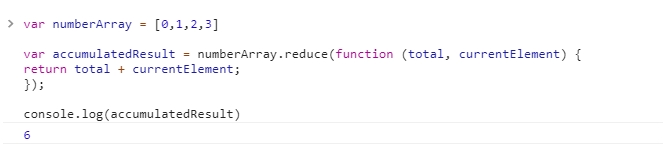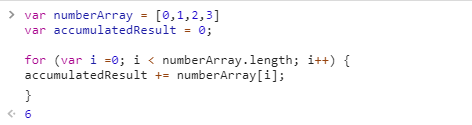# How to Use Reduce on JavaScript Arrays

Sharing is Caring

In functional programming, being able to reduce is one of the fundamental concepts.

Reduce is used to iterate through the values in an array and condense all of the values into a single value.

Usually the value is a sum of all of the elements, but it could be used to decrement, multiply or even divide.

## What is Reduce used for?

Normally, you would want to use a reduce method when you want to sum all of the values in an array and then use the total for some operation. For example, it might make sense to use a reducer when you want to get the total number of items in a shopping cart and determine the tax or determine how many items a customer purchased.

I like using reducers to avoid having to write boiler plate code for loops and being able to do really simple one line operations.

## How to Use Reduce

A reduce method takes in a function as a parameter, and that function needs to take in an accumulator and the current element.

So, basically, a really simple example of how to use reduce is this:

```var numberArray = [0,1,2,3]

var accumulatedResult = numberArray.reduce(function (total, currentElement) {
});

```

If we ran this in the developer console of Chrome, we would see this:As I mentioned, every element of the array is added together and then the total is available in the accumulatedResult variable. We could write this as a for loop and get exactly the same result if we wanted to but it would be more code:

```var numberArray = [0,1,2,3]
var accumulatedResult = 0;

for (var i = 0; i &amp;lt; numberArray.length; i++) {
accumulatedResult += numberArray[i];
}

```

And, if we ran this for code for a loop in the developer console, we would see this:So this means, that a reduce method cycles through the values in an array – in a way similar to a for loop but it’s able to do some mathematical operations like summing of values and store a lot less variables in code and use a lot less boiler plate.

## Advantages of JavaScript’s Reduce Method

• the reduce method is that it can handle array elements that don’t have a value. Technically the for loop above would crash if there was a null or undefined value.
• Reduce methods are usually much shorter – and much easier to understand.
• Reduce methods can be chained together – forEach and for loops can’t be easily which means they can’t really be used too well in functional programming.

Also published on Medium.

Sharing is Caring